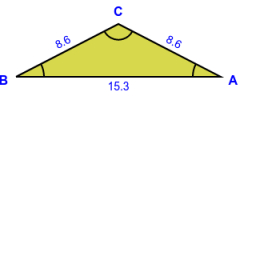# Isosceles 71154

Calculate all interior angles in the isosceles triangle ABC if we know that BC is the base, and we also know: | ∢BAC | = α; | CABCA | = 4α

α =  20 °
β =  80 °
γ =  80 °

### Step-by-step explanation:Did you find an error or inaccuracy? Feel free to write us. Thank you!

Tips for related online calculators
Check out our ratio calculator.
Do you have a linear equation or system of equations and looking for its solution? Or do you have a quadratic equation?